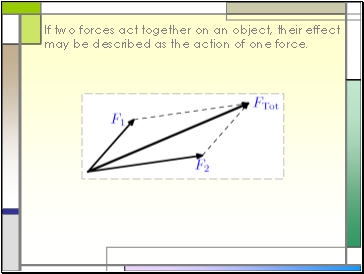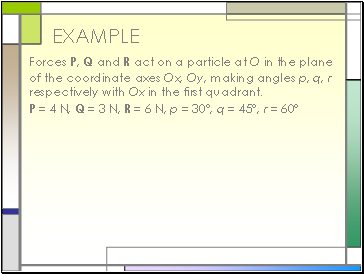# Resultant forcesPage 1

#### WATCH ALL SLIDES

Slide 1## Resultant Forces

Slide 2If two forces act together on an object, their effect may be described as the action of one force.

Slide 3## Example

Two forces of magnitude 5N and 6N act on a particle. They act at right angles. Find the magnitude and direction of their resultant.

Slide 4EXAMPLE

Two forces acting on a particle have magnitudes 8N and 3N. The angle between their directions is 60°. Find the resultant force acting on the particle.

Slide 5EXAMPLE

Three forces (2i – j) N, 3i N and (-i + 4j) N, where i and j are unit vectors due east and due north respectively, act on a particle. Find the magnitude and direction of the resulting force.

Slide 6EXAMPLE

Forces P, Q and R act on a particle at O in the plane of the coordinate axes Ox, Oy, making angles p, q, r respectively with Ox in the first quadrant.

P = 4 N, Q = 3 N, R = 6 N, p = 30°, q = 45°, r = 60°